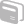# Activities

••• ##### Subject Area

• Math: Algebra I: Equations and Inequalities
• Math: Algebra I: Expressions

• ##### Author6-8

60 Minutes

• ##### Device
•TI-Nspire™ CX/CX II
•TI-Nspire™ CX CAS/CX II CAS
• TI-Nspire™
• TI-Nspire™ CAS
• ##### Software

TI-Nspire™
TI-Nspire™ CAS

3.1

## TI-PoW: Caliyah's Cart

#### Activity Overview

This problem presents an opportunity for students to think about tables and formulas, as well as linear relationships, systems of inequalities, and liner programming. If your state has adopted the Common Core State Standards, this alignment might be helpful: Algebra: Represent Equations and Inequalities A.REI.12. Graph the solutions to a linear inequality in two variables as a halfplane (excluding the boundary in the case of a strict inequality), and graph the solution set to a system of linear inequalities in two variables as the intersection of the corresponding half-planes. Grade 7: Expressions & Equations Solve real-life and mathematical problems using numerical and algebraic expressions and equations. Grade 8: Expressions & Equations Analyze and solve linear equations and pairs of simultaneous linear equations.